WIENER FILTERING

# Wiener Filtering

### Theory

The inverse filtering is a restoration technique for deconvolution, i.e., when the image is blurred by a known lowpass filter, it is possible to recover the image by inverse filtering or generalized inverse filtering. However, inverse filtering is very sensitive to additive noise. The approach of reducing one degradation at a time allows us to develop a restoration algorithm for each type of degradation and simply combine them. The Wiener filtering executes an optimal tradeoff between inverse filtering and noise smoothing. It removes the additive noise and inverts the blurring simultaneously.

The Wiener filtering is optimal in terms of the mean square error. In other words, it minimizes the overall mean square error in the process of inverse filtering and noise smoothing. The Wiener filtering is a linear estimation of the original image. The approach is based on a stochastic framework. The orthogonality principle implies that the Wiener filter in Fourier domain can be expressed as follows: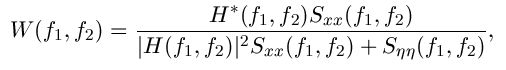where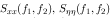are respectively power spectra of the original image and the additive noise, and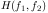is the blurring filter. It is easy to see that the Wiener filter has two separate part, an inverse filtering part and a noise smoothing part. It not only performs the deconvolution by inverse filtering (highpass filtering) but also removes the noise with a compression operation (lowpass filtering).

### Implementation

To implement the Wiener filter in practice we have to estimate the power spectra of the original image and the additive noise. For white additive noise the power spectrum is equal to the variance of the noise. To estimate the power spectrum of the original image many methods can be used. A direct estimate is the periodogram estimate of the power spectrum computed from the observation: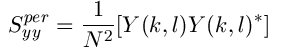where Y(k,l) is the DFT of the observation. The advantage of the estimate is that it can be implemented very easily without worrying about the singularity of the inverse filtering. Another estimate which leads to a cascade implementation of the inverse filtering and the noise smoothing is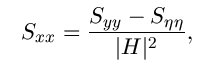which is a straightforward result of the fact: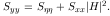The power spectrum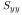can be estimated directly from the observation using the periodogram estimate. This estimate results in a cascade implementation of inverse filtering and noise smoothing: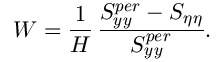The disadvantage of this implementation is that when the inverse filter is singular, we have to use the generalized inverse filtering. People also suggest the power spectrum of the original image can be estimated based on a model such as the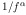model.

### Experimental Result

To illustrate the Wiener filtering in image restoration we use the standard 256x256 Lena test image. We blur the image with the lowpass filter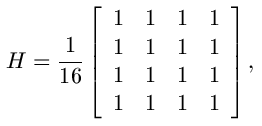then put into the blurred image the additive white Gaussian noise of variance 100. The Wiener filtering is applied to the image with a cascade implementation of the noise smoothing and inverse filtering. The images are listed as follows together with the PSNRs and MSEs. Notice that the restored image is improved in terms of the visual performance, but the MSEs don't indicate this, the reason of which is that MSE is not a good metric for deconvolution.

#### Test Image Lena and Blurred Image LenaStandard Lena Image PSNR = Infinity, MSE = Zero Blurred Lena Image PSNR = 23.2993, MSE = 304.1938 Restored Lena Image PSNR = 19.1447, MSE = 791.7906